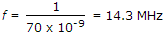# Electronics and Communication Engineering - Digital Electronics

31.

A multiple emitter transistor has many emitters and collectors.

 A. True B. False

Answer: Option B

Explanation:

It has many emitters but one collector.

32.

A mode-10 counter can divide the clock frequency by a factor of

 A. 10 B. 100 C. 1000 D. 10000

Answer: Option A

Explanation:

Decade counter is divide by 10 counter.

33.

Which of the following binary numbers is equivalent to decimal 10?

 A. 1000 B. 1100 C. 1010 D. 1001

Answer: Option C

Explanation:

1010 = 8 + 0 + 2 + 0 = 10.

34.

A 4 bit synchronous counter has flip flops having propagation delay of 50 ns each and AND gates having propagation delay of 20 ns each. The maximum frequency of clock pulses can be

 A. 20 MHz B. 50 MHz C. 14.3 MHz D. 5 MHz

Answer: Option C

Explanation:

Maximum delay = 50 + 20 = 70 x 10-9 s.

Hence.

35.

A counter has 4 flip flops. It divides the input frequency by

 A. 4 B. 2 C. 8 D. 16

Answer: Option D

Explanation:

24 = 16.

#### Current Affairs 2021

Interview Questions and Answers